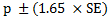# What is Statistical Confidence?

## Understand Statistical Confidence

The A/B experiment report uses Unique Visitors and Goals to calculate the Conversion Rate, Improvement and Confidence with a Statistical Significance.

If you go to your Experience Report, you will find these metrics:Let's see what these columns mean:

• Conversion Rate: this column shows the percentage of visitors that turned into conversions as well as the error interval;
• Best Improvement: this column reports the percentage change of the variation compared to the Control;
• Confidence: this column reports the significance, or how different the confidence interval for the conversion rate for the experiment variation is when compared to the control/original variation (this must be at least 97% confident before being marked as a winner). If the confidence is not showing any number, this is because (by default) there is a minimum of 5 conversions needed for each variation in order to calculate it. It also must meet the minimum visitors set for each variation.  If you have changed the minimum conversions then the minimum you chose would have to be met.  The gray/green dots in that column indicate:
• 1 green dot for 75%-85% confidence
• 2 green dots for 85%-95% confidence
• 3 green dots for 95%-96% confidence
• 4 green dots for 96%-97% confidence
• 5 green dots for 97% and above
• Conversions / Visitors: this column reports the number of conversions received and the number of unique visitors that saw the specific variation;

## Two-Tailed

We at Convert decided to use 2-tailed Z-test at a .05 confidence level (95%) (that is .025 for each tail being a normal symmetric distribution) with the option to change this between 95%-99%.

## Calculations

The values used in the Experience Report are calculated as noted below.

### Conversion Rate

For each variation the Conversion Rate is calculated as "(Total Number of Goal Conversions / Number of Unique Visitors) * 100"### Conversion Rate Change for Variations

The percentage change of the conversion rate between the experiment variation and the original/control variation:### Confidence Intervals

A statistical method for calculating a confidence interval around the conversion rate is used for each variation. The standard error (for 1 standard deviation) is calculated using the Wald method for a binomial distribution. Thus, for a given conversion rate (p) and sample size (number of Unique Visitors), standard error is calculated as:This formula is one of the simplest formulas used to calculate standard error and assumes that the binomial distribution can be approximated with a normal distribution (because of the central limit theorem) http://en.wikipedia.org/wiki/Binomial_proportion_confidence_interval. The sample distribution can be approximated with a normal distribution when there are more than 10 conversions on the specific goal.

To determine the confidence interval for the conversion rate multiply the standard error with the 95th percentile of a standard normal distribution (a constant value equal to 1.65).

In other words, you can be sure with 90% confidence that your true conversion rate p lies within this range:For 95% confidence use p ± (1.96 * SE), while for 99% confidence use p ± (2.575 * SE).

### Winner

To determine whether the results are significant (that the conversion rates for each variation are not different because of random variations), a ZScore is calculated as follows:The ZScore is the number of standard deviations between the control and test variation mean values, described at http://en.wikipedia.org/wiki/Zscore. Using a standard normal distribution the 95% significance is determined when the view event count is greater than 1000 and one of the following criteria is met:

• Probability(ZScore) > 95%

• Probability(ZScore) < 5%

### Improvement

The chance to be different (displayed on the report) is derived from the Probability(ZScore) value where:

• If Probability(ZScore) <= 0.5 then
Improvement = 1- Probability(ZScore)
• If Probability(ZScore) > 0.5 then
Improvement = Probability(ZScore)Want to try out the advanced audience targeting in Convert Experiences? Give it a free 15-day try here.